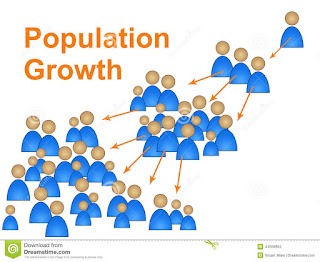## Wednesday, February 3, 2016

### When is the world going to be full?A possible project which could be used in a math class.  I have designed this to fit in the Alberta Math 30-2 curriculum.  Feel free to change or alter as you see fit.

Pose the question:

Introduction: When is the world going to be full?
Give students 5 minutes to talk in groups.  After, have a debrief and share possible hypotheses.

Next, ask for possible information we will need to answer this question more accurately.  Some other possible questions might be

• How many people can fit on the plant?
• How many people can the plant sustain? (Different question than the first)
• What is our current growth rate?
• What is our current population?
Some of these questions will be easy to solve, while others might be more difficult.  I would ask students, in groups, to research the answers to the questions they asked.  Some of these will have an answer all will agree with, such as "What is our current population?", while other questions might have a range of answers depending on the website found, such as "How many people can the planet sustain?"  Create answers that everyone in the class can agree with, or even commons answers in different groups as long as each student in each group agrees.

Body:
Creating an extrapolation of our population.

You can google our current growth rate, however this number, most likely, has no meaning or even understanding of how it was derived.  As a class, possible growth rates will be explored.  Below is a chart of recent population numbers on our planet.  (You might want to use different years, or more years).

 Year Population (Billion) 2016 7.4 2015 7.3 2010 6.9 2005 6.5 2000 6.1 1995 5.7 1990 5.3 1985 4.9 1980 4.4 1975 4.0 1970 3.7 1965 3.3 1960 3 1955 2.8

Now the question becomes, "What sort of data is this?"  This is when I would pause and teach exponential, sinusoidal, linear, quadratic, cubic, and logarithmic functions.  Using this data, I would create an equation of each type of function.  Here is a graph of all the functions displayed together...(I used 1970 as year 0.  This occurred as, after deciding this, I needed more data points. I also added a logistic curve to show what would happen if population growth become 0)

Now is the discussion time.....

Ask some general questions such as:
• Any general thoughts?
• Any similarities?
• Could you create a possible scenario in which each graph would be accurate?
You could also embed the critical learning of each function into this:  For example you could ask:
• What is the amplitude, period, and median value for the sine function?  What does that mean in this context?
• What are the zeros of the cubic function, what does this mean in this context?
• What is the growth rate of the exponential function?  What does this mean in this context?
And any other questions which may come to mind.  Now you may want to remove any functions in which the class agrees might be inaccurate.  Such as the cubic, sinusoidal, and/or linear.

Using the remaining graphs determine when the Earth would be full, using the number the class researched earlier.  The answers might be earlier, or later, than the class first hypothesized.

Conclusion:
Have the discussion "Should population growth be addressed? Why or why not?"

Do not rush this project, take your time.  Stop, throughout, to teach certain skills, or concepts.  Also allow students to research on their own and pose their own questions throughout.